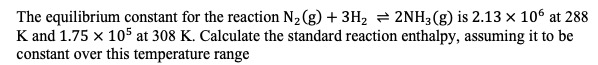# The equilibrium constant for the reaction N2 (g) 3H2 2NH3(g) is 2.13 x 106 at 288K and 1.75 x 105 at 308 K. Calculate the standard reaction enthalpy, assuming it to beconstant over this temperature range

Question
50 viewshelp_outlineImage TranscriptioncloseThe equilibrium constant for the reaction N2 (g) 3H2 2NH3(g) is 2.13 x 106 at 288 K and 1.75 x 105 at 308 K. Calculate the standard reaction enthalpy, assuming it to be constant over this temperature range fullscreen
check_circle

Step 1

Given:

Temperature T1 = 288 K.

Temperature T2 = 308 K.

Equilibrium Constant at T1 (K1) = 2.13×106.

Equilibrium Constant at T2 (K2) = 1.75×105.

Step 2

Van`t hoff equation ...

### Want to see the full answer?

See Solution

#### Want to see this answer and more?

Solutions are written by subject experts who are available 24/7. Questions are typically answered within 1 hour.*

See Solution
*Response times may vary by subject and question.
Tagged in

### Chemistry